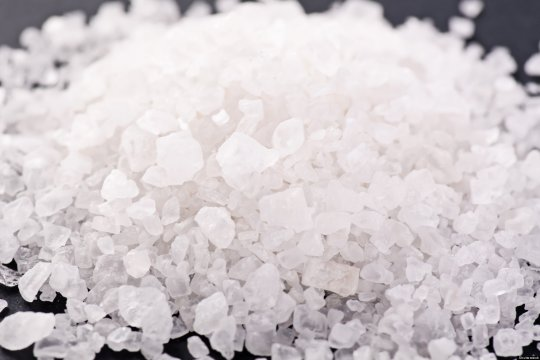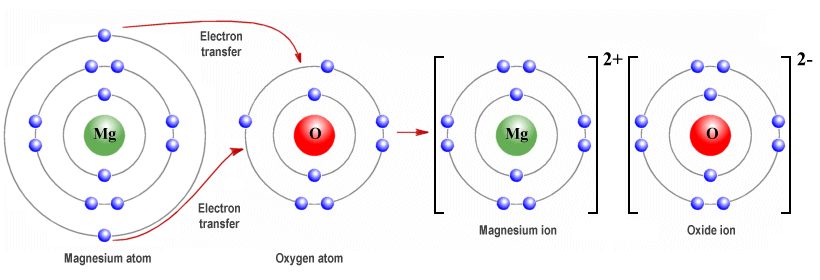Ionic Solids

Ionic solids are composed of oppositely charged ions (positively charged cations and negatively charged anions). They are held together by electrostatic forces in a continuous three-dimensional network in which the ions are arranged in such a way that electrostatic attraction between opposite charges is maximised, and electrostatic repulsion between like charges is minimised.

One of the ionic solids you will be familiar with is sodium chloride (or common salt) without which we could not live. Sodium chloride forms when the single electron from the valence shell of a sodium atom is transferred to the valence shell of a chlorine atom. Both atoms achieve a full outer electron shell as a consequence, but become ionised in the process. The sodium atoms acquire a positive charge and the chlorine atoms acquire a negative charge.Sodium chloride (common salt) crystals - salt is an ionic solid

It is the electrostatic attractions between these positively and negatively charged ions that holds the crystalline structure of an ionic solid together. Like network covalent solids, ionic solids tend to be hard and brittle and have high melting and boiling points because of the strength of the bonds holding the atoms together within the crystal lattice. It takes a lot of energy to break those bonds down. Having said that, ionic bonds are generally not as strong as covalent bonds.

Each ion in an ionic solid is surrounded by ions of the opposite charge in a three-dimensional crystal lattice. In sodium chloride, for example, each sodium ion (Na +) is surrounded by six chloride (Cl -) ions. Similarly, each chloride ion is surrounded by six sodium ions. In another ionic solid, calcium fluoride (CaF2 ), each calcium ion (Ca +) is surrounded by eight fluoride (F -) ions, while each fluoride ion is surrounded by four calcium ions.

The number of oppositely charged ions surrounding an ion in an ionic solid is called its coordination number. Thus, in sodium chloride, the coordination number of both Na+ and Cl- is six (6). In calcium fluoride, the coordination number of Ca+ is eight (8), while the coordination number of F - is four (4).

The main differences between the various ionic compounds, in terms of physical properties such as melting and boiling points, depends on the strength of the ionic bonds holding them together. This will depend chiefly on two things: the number of charges on each ion, and the size of the ions.

Magnesium oxide (MgO), for example, has exactly the same crystalline structure as sodium chloride, but each magnesium atom donates two (2) valence electrons to each oxygen atom, resulting in magnesium ions (Mg 2+) that have twice the positive charge of sodium ions, and oxide (O -2) ions that have double the negative charge of chloride ions.In magnesium oxide, each magnesium atom donates two electrons to an oxygen atom

The electrostatic attraction between magnesium ions and oxide ions is thus much greater than the electrostatic attraction between sodium ions and chloride ions. This means that the bonds are stronger and more difficult to break, resulting in much higher melting and boiling points for magnesium oxide (2825 °C and 3600 °C respectively) than for sodium chloride (801 °C and 1413 °C).

The size of the ions is important because smaller ions tend to be closer together, which means that the electrostatic attractions between each ion are greater. Rubidium iodide (RbI), for example, has lower melting and boiling points than sodium chloride because both the rubidium ions (Rb +) and the iodide (I -) ions are bigger than sodium and chloride ions.

The precise melting and boiling points of an ionic solid will depend on the lattice energy of the ionic solid. Lattice energy can be defined in two different ways. One definition describes the lattice energy as the energy needed to break an ionic solid apart and turn its constituent atoms into gaseous ions. This definition always gives a positive value for lattice energy, because the process is an endothermic reaction (i.e. a reaction that requires heat energy in order for it to take place).

The alternative definition is that lattice energy is the heat energy produced by the opposite process, i.e. the energy released when gaseous ions bond together to form an ionic solid. A reaction in which heat is given off is called an exothermic reaction. The lattic energy will have a negative value when this definition is used, because heat energy has been lost.

Whichever definition we use, it is the magnitude of the lattice energy that is important. When we talk about lattice energy in the context of ionic solids, therefore, we think in terms of absolute values. Essentially, the greater the lattice energy of of an ionic compound is, the higher the melting and boiling points of that compound will be.

In terms of their electrical behaviour, ionic solids are effectively insulating materials. The valence shell of each atom in an ionic solid is complete, because the atom has either lost or gained the requisite number of electrons. It is therefore very difficult for electric current to flow in ionic solids, because there are no free electrons in the crystalline structure. An ionic solid in solution is a different matter.

You probably know that salt (sodium chloride) dissolves in water, but why is that? The reason is that water molecules are polar. Each water molecule consists of two hydrogen atoms covalently bonded to one oxygen atom. Each hydrogen atom's electron strongly attracted to the highly electronegative oxygen atom, and spends far more time around the nucleus of the oxygen atom than around the nucleus of the hydrogen atom.

Each hydrogen atom carries a net positive charge, while the oxygen atom carries a net negative charge. This effectively turns the water molecule into a dipole - one end of the molecule (the oxygen atom) has a small negative charge, and the other end (the hydrogen atoms) has a small positive charge. The positively charged sodium ions are attracted to the negatively charged oxygen atoms, while the negatively charged chloride ions are attracted to the to positively hydrogen atoms.

These attractions are strong enough to overcome the electrostatic forces holding the sodium and chloride ions together, and the sodium chloride dissociates (i.e. splits into its constituent ions). Once the sodium and chloride ions are separated, they can move around freely in the water, which allows the solution to conduct electrical current.

The quantity of a particular ionic solid that can be dissolved in a given amount of a polar solvent (like water) will depend on the lattice energy of the ionic solid. The lower the lattice energy, the greater the quantity of the ionic solid that can be dissolved.

The final point to make here about ionic solids relates to their tendency to be brittle. An ionic solid may be hard, but if enough force is applied to an ionic crystal, the ion layers can be shifted relative to one another. If the degree of shift is great enough, ions having the same charge may be brought into close proximity with one another. These like charges repel each other, creating a fracture in the material.